>  CHEMISTRY TEST 2

# CHEMISTRY TEST 2

Test Description

## 30 Questions MCQ Test | CHEMISTRY TEST 2

 1 Crore+ students have signed up on EduRev. Have you?
CHEMISTRY TEST 2 - Question 1

### 1 mole of ferric oxalate is oxidised by x mole of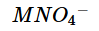in acidic medium. Hence value of x is

CHEMISTRY TEST 2 - Question 2

### In the reaction,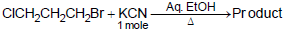CHEMISTRY TEST 2 - Question 3

### 1-Phenyl ethyl chloride undergoes alkaline hydrolysis to give 1-phenyl ethanol with

CHEMISTRY TEST 2 - Question 4

The relative strength of boron trihalides as electron pair acceptors increases in the order as

CHEMISTRY TEST 2 - Question 5

Which trihalide is not hydrolysed ?

CHEMISTRY TEST 2 - Question 6

Which does not form precipitate with AgNO3 ?

CHEMISTRY TEST 2 - Question 7

When ammonia is added to green aqueous solution of nickel (II) sulphate,the colour of the solution changes to blue violet. This is caused by

CHEMISTRY TEST 2 - Question 8

The correct IUPAC name of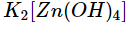CHEMISTRY TEST 2 - Question 9

What is the maximum number of hydrogen bonds in which a water molecule can participate ?

CHEMISTRY TEST 2 - Question 10

Solubility of a salt A2B3 is 1x10-4 mol L-1 , itssolubility product is

CHEMISTRY TEST 2 - Question 11

If ammonia is added to pure water, the concentration of a chemical species already present will decrease. The species is

CHEMISTRY TEST 2 - Question 12

20 cm3 of xM solution of HCl is exactly neutralised by 40 cm3 or 0.5 M NaOH solution, the molarity of HCl solution is

CHEMISTRY TEST 2 - Question 13

In the given transformation, which of the following is the most appropriate reagent ?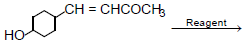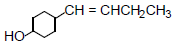CHEMISTRY TEST 2 - Question 14

Under what conditions of temperature and pressure the formation of atomic hydrogen from molecular hydrogen will be favoured most ?

CHEMISTRY TEST 2 - Question 15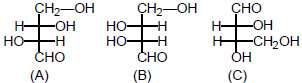(D) and (L) Configuration of above carbohydrate is

CHEMISTRY TEST 2 - Question 16

What are X and Y in the following reaction sequence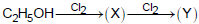CHEMISTRY TEST 2 - Question 17

Copper sulphate solution reacts with KCN to give

CHEMISTRY TEST 2 - Question 18

Bakelite is obtained from phenol by reacting with

CHEMISTRY TEST 2 - Question 19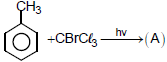(no ring substitution) Product (A) is

CHEMISTRY TEST 2 - Question 20

The energy required to break one mole of Cl—Cl bonds in Cl2 is 242 kJ mol-1. The longest wavelength of light capable of breaking single Cl—Cl bond is
(c = 3 x 108 m sec-1, NA = 6.023 x 1023 mol-1)

CHEMISTRY TEST 2 - Question 21

Acetamide reacts with NaOBr in alkaline medium to form

CHEMISTRY TEST 2 - Question 22

A 0.6% urea solution is isotonic with

CHEMISTRY TEST 2 - Question 23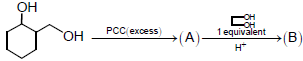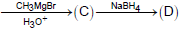Product (D) will be

CHEMISTRY TEST 2 - Question 24

Identify the statement which is not correct regarding copper sulphate

CHEMISTRY TEST 2 - Question 25

What takes place when zinc metal is added to an aqueous solution containing magnesium nitrate and silver nitrate ?

1. Zn is oxidised
2. Mg2+ is reduced
3. Ag+ is reduced
4. No reaction

CHEMISTRY TEST 2 - Question 26

Consider the reaction, Cl2 (g) + 2Br- (aq) → Br2 (g) + 2Cl- (aq).The emf of the cell, when [Cl-] = [Br2] = [Br-] = 0.01 M and Cl2 gas is at 1 atm pressure, will be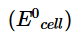=0.29V

CHEMISTRY TEST 2 - Question 27

Which of the following statements is not true about potash alum ?

CHEMISTRY TEST 2 - Question 28

For the process, NH3 (g) + HCl(g) → NH4Cl(s);

CHEMISTRY TEST 2 - Question 29

Which of the following reaction sequences would be the best for synthesis of t-butyl alcohol ?

CHEMISTRY TEST 2 - Question 30

The half life period of a first order reaction is 10 minutes. The time required for the concentration of reactant to change form 0.08 M to 0.02 M is

 Use Code STAYHOME200 and get INR 200 additional OFF Use Coupon Code